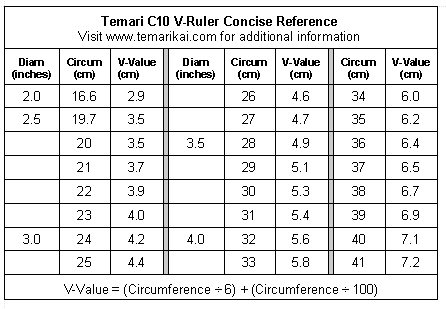#V-Ruler Reference Chart

This info remains on TemariKai.com due to V-Rulers being in use for so many years; however they are no longer available and have been replaced with C-10 Rulers.

As referenced in how to use a V-ruler for dividing a 10 Combination temari, one need not carry around the mathematical formula for calculating the centers of this division. to review, it is an easy three-step process: measure the circumference of the mari in cm; divide that by 6, and remember that result (call it Result A). Then, divide the original circumference by 100 and remember that result (call it Result B).  Add Result A and Result B together, and you have the distance between centers for that particular mari. As a formula, it is written as (circum/6)+(circum/100) = distance between C10 centers (and yes, that circumference/100 is very important. One may think it too small to worry about, but it's not and the symmetry will not work without it).

Using a V-ruler is a quicker and easier method since one only needs the value of the circumference, and a V-ruler reference chart, commonly found in many English and Japanese references: